# Spherical polar coordinates

In mathematics and physics, spherical polar coordinates (also known as spherical coordinates) form a coordinate system for the three-dimensional real space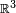$\mathbb{R}^3$. Three numbers, two angles and a length specify any point in$\mathbb{R}^3$. The two angles specify the position on the surface of a sphere and the length gives the radius of the sphere.

Spherical polar coordinates are useful in cases where there is (approximate) spherical symmetry, in interactions or in boundary conditions (or in both). In such cases spherical polar coordinates often allow the separation of variables simplifying the solution of partial differential equations and the evaluation of three-dimensional integrals.

The practice of specifying locations on earth using latitude, longitude and altitude is a version of spherical polar coordinates.

## Definition

Let x, y, z be Cartesian coordinates of a vector$\vec\mathbf{r}$ in$\mathbb{R}^3$, that is,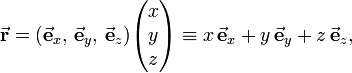$\vec\mathbf{r} = (\vec\mathbf{e}_x, \, \vec\mathbf{e}_y, \, \vec\mathbf{e}_z) \begin{pmatrix} x\\y\\z\\ \end{pmatrix} \equiv x\,\vec\mathbf{e}_x + y\,\vec\mathbf{e}_y + z\, \vec\mathbf{e}_z,$

where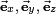$\vec\mathbf{e}_x, \, \vec\mathbf{e}_y, \, \vec\mathbf{e}_z$ are unit vectors along the x, y, and z axis, respectively. The x, y, and z axes are orthogonal and so are the unit vectors along them.

The length r of the vector$\vec\mathbf{r}$ is one of the three numbers necessary to give the position of the vector in three-dimensional space. By applying twice the theorem of Pythagoras we find that r2 = x2 + y2 + z2.

Let θ be the colatitude angle (see the figure) of the vector$\vec\mathbf{r}$. In the usual system to describe a position on Earth, latitude has its zero at the equator, while the colatitude angle, introduced here, has its zero at the "North Pole". That is, the angle θ is zero when$\vec\mathbf{r}$ is along the positive z-axis. The sum of latitude and colatitude of a point is 900; these angles being complementary explains the name of the latter. The colatitude angle is also called polar or zenith angle in the literature.

The angle φ gives the angle with the x-axis of the projection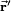$\vec\mathbf{r}'$ of$\vec\mathbf{r}$ on the x-y plane. The angle φ is the longitude angle (also known as the azimuth angle).

Note that the projection$\vec\mathbf{r}'$ has length r sinθ. The length of the projection of$\vec\mathbf{r}'$ on the x and y axis is therefore r sinθcosφ and r sinθsinφ, respectively. In summary, the spherical polar coordinates r, θ, and φ of$\vec\mathbf{r}$ are related to its Cartesian coordinates by\begin{align} x &= r \sin\theta\cos\phi \\ y &= r \sin\theta\sin\phi \\ z &= r \cos\theta \end{align}

Given a spherical polar triplet (r, θ, φ) the corresponding Cartesian coordinates are readily obtained by application of these defining equations.

The figure makes clear that 00 ≤ φ ≤ 3600, 00 ≤ θ ≤ 1800, and r > 0. The coordinate surfaces are:

1. r constant, all θ and φ: surface of sphere.
2. θ constant, all r and φ: surface of a cone.
3. φ constant, all r and θ: plane.

The computation of spherical polar coordinates from Cartesian coordinates is somewhat more difficult than the converse, due to the fact that the spherical polar coordinate system has singularities, also known as points of indeterminacy. The first such point is immediately clear: if r = 0, we have a zero vector (a point in the origin). Then θ and φ are undetermined, that is to say, any values for these two parameters will give the correct result x = y = z = 0. Compare this to the case that one of the Cartesian coordinates is zero, say x = 0, then the other two coordinates are still determined (they fix a point in the yz-plane). Two other points of indeterminacy are the "North" and the "South Pole", θ = 00 and θ = 1800, respectively (while r ≠ 0). On both poles the longitudinal angle φ is undetermined.

So, when going from Cartesian coordinates to spherical polar coordinates, one has to watch for the singularities, especially when the transformation is performed by a computer program. Given x, y and z, the consecutive steps are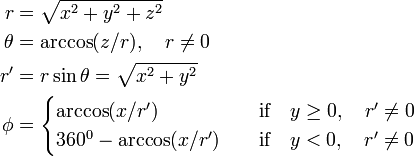\begin{align} r &= \sqrt{x^2+y^2+z^2} \\ \theta &= \arccos(z/r), \quad r \ne 0 \\ r' & = r \sin\theta = \sqrt{x^2+y^2} \\ \phi & = \begin{cases} \arccos(x/r')&\quad \hbox{if}\quad y \ge 0,\quad r' \ne 0 \\ 360^0 - \arccos(x/r')&\quad \hbox{if}\quad y < 0,\quad r'\ne 0 \\ \end{cases} \end{align}

### Latitude, longitude, and altitude

Locations on earth are often specified using latitude, longitude and altitude. These values correspond to the spherical polar coordinates introduced in this article, with some differences, however.

Latitude λ is conventionally measured as angles north and south of the equator, with latitudes north of the equator taken as positive, and south taken as negative. Values of latitude are always within the range −90° to +90°. This corresponds to the polar angle measured from the z-axis, except that latitude is measured from the equator (the x-y-plane). The angles θ and λ are complementary, i.e., their sum is 90°.

The geographical coordinate longitude φg (the suffix g is added to distinguish it from the polar coordinate φ) is measured as angles east and west of the prime meridian, an arbitrary great circle passing through the z-axis. Longitude is measured as the angular distance in the closest direction from the prime meridian, or, alternatively, as a positive angle to the east (counterclockwise when looking from the North Pole towards the center of the earth) and negative to the west. Longitude is always within the range −180° to +180°.

Altitude is measured from the surface— called mean sea level—of a hypothetical spheroid, or other datum, and corresponds to the polar coordinate r, with the radius of the earth subtracted. Since the earth is not a perfect sphere, neither its center nor its radius are defined in a unique manner, but for the sake of argument we ignore this and introduce rearth as the fictive radius of the earth.

Conversion from latitude λ, longitude φg, and altitude h to spherical polar coordinates is as follows: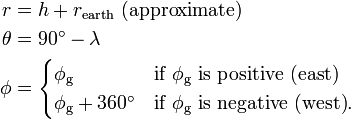\begin{align} r &= h + r_\mathrm{earth} \mbox{ (approximate)}\\ \theta &= 90^\circ - \lambda \\ \phi &= \begin{cases} \phi_\mathrm{g} & \mbox{if }\phi_\textrm{g} \mbox{ is positive (east)} \\ \phi_\mathrm{g} +360^\circ & \mbox{if }\phi_\textrm{g} \mbox{ is negative (west)}. \end{cases} \end{align}

#### Examples$\lambda = 40.7^\circ \quad \hbox{and}\quad \phi_\mathrm{g} = -74.017^\circ$
so that the polar coordinates of New York city are$\theta = 49.3^\circ \quad \hbox{and}\quad \phi = 285.983^\circ$
• Melbourne is at 37° 49' S, 144° 58' E, this means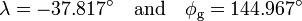$\lambda = -37.817^\circ \quad \hbox{and}\quad \phi_\mathrm{g} = 144.967^\circ$
so that the polar coordinates of Melbourne are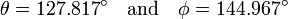$\theta = 127.817^\circ \quad \hbox{and}\quad \phi = 144.967^\circ$

## Other convention

The notational convention introduced above (θ for the colatitude angle, φ for the azimuth angle) is used universally in physics. It is in accordance with advice of the International Standards Organization (ISO 31-11, which, however, advises the use of ρ instead of r ). In mathematics—especially in the older and the European literature—the convention is very widespread, too. To quote a few prestiguous mathematical books that apply it: Abramowitz and Stegun (p. 332), Whittaker and Watson (p. 391), Courant and Hilbert (p.195), and Kline (p. 527). Until the 1960s this convention was used universally, also in mathematical textbooks, see e.g. the 1959 edition of Spiegel (p. 138).

Somewhere in the 1960s it became custom in American mathematical textbooks to use a notation in which φ and θ are interchanged, see e.g. Kay (p. 24) and Apostol (p. 419). This was done in order to not confuse students by changing the meaning of the Greek letter θ in the transition from 2D to 3D polar coordinates, as can be gathered from the following quotation of Eric Weisstein:

In this work, following the mathematics convention, the symbols for the radial, azimuth, and zenith coordinates are taken as r, θ, and φ, respectively. Note that this definition provides a logical extension of the usual polar coordinates notation, with θ remaining the angle in the xy-plane and φ becoming the angle out of that plane. The sole exception to this convention in this work is in spherical harmonics, where the convention used in the physics literature is retained (resulting, it is hoped, in a bit less confusion than a foolish rigorous consistency might engender).

In more advanced treatises—also American—on spherical functions the old convention remains in use, see e.g. Miller (p. 164). The swapping of θ and φ can only be called unfortunate, because it meant a break with the huge existing mathematics and physics literature covering more than a century, and since there exists an obvious pedagogical alternative, namely, calling the angle, which appears in the 2D polar coordinates, φ instead of θ.

The notation, in which the angle between the vector r and the z-axis is φ, is followed by the Maple algebraic program package and also by the numerical package Matlab. (Matlab also redefines the zero of φ to be on the equator, which makes φ a latitude angle). The Mathematica package follows the convention that has θ as the angle between the vector and the z-axis.

## Unit vectorsUnit vectors.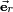$\vec{\mathbf{e}}_r$ is perpendicular to the surface of the sphere, while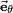$\vec{\mathbf{e}}_\theta$ and$\vec{\mathbf{e}}_\phi$ are tangent to the surface.

We will define algebraically the orthogonal set (a coordinate frame) of spherical polar unit vectors depicted in the figure on the right. In doing this, we first wish to point out that the spherical polar angles can be seen as two of the three Euler angles that describe any rotation of$\mathbb{R}^3$.

Indeed, start with a vector along the z-axis, rotate it around the z-axis over an angle φ. Perform the same rotation on the x, y, z coordinate frame. This rotates the x- and y-axis over a positive angle φ. The y-axis goes to the y'-axis. Rotate then the vector and the new frame over an angle θ around the y'-axis. The vector that was initially on the z-axis is now a vector with spherical polar angles θ and φ with respect to the original (unrotated) frame. Expressed in equation form this reads,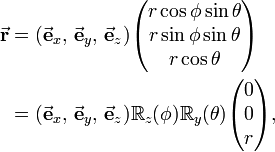\begin{align} \vec\mathbf{r} &= (\vec\mathbf{e}_x, \, \vec\mathbf{e}_y, \, \vec\mathbf{e}_z) \begin{pmatrix} r\cos\phi\sin\theta \\ r\sin\phi\sin\theta \\ r\cos\theta \\ \end{pmatrix} \\ &= (\vec\mathbf{e}_x, \, \vec\mathbf{e}_y, \, \vec\mathbf{e}_z) \mathbb{R}_z(\phi) \mathbb{R}_y(\theta) \begin{pmatrix} 0 \\ 0 \\ r \\ \end{pmatrix}, \end{align}

where the two rotation matrices are defined by$\mathbb{R}_z(\phi) \equiv \begin{pmatrix} \cos\phi & - \sin\phi & 0 \\ \sin\phi & \cos\phi & 0 \\ 0 & 0 & 1 \\ \end{pmatrix}, \qquad \mathbb{R}_y(\theta) \equiv \begin{pmatrix} \cos\theta & 0 & \sin\theta \\ 0 & 1 & 0 \\ -\sin\theta & 0 & \cos\theta \\ \end{pmatrix}.$

By direct matrix multiplication the matrix expression for the spherical polar coordinates of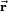$\vec{\mathbf{r}}$ is easily verified—it could have been postulated without reference to Euler rotations and proved by verification.

We now introduce the coordinate frame depicted in the figure on the right: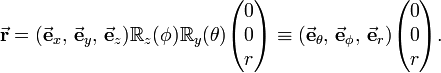\begin{align} \vec\mathbf{r} &= (\vec\mathbf{e}_x, \, \vec\mathbf{e}_y, \, \vec\mathbf{e}_z) \mathbb{R}_z(\phi) \mathbb{R}_y(\theta) \begin{pmatrix} 0 \\ 0 \\ r \\ \end{pmatrix} \equiv (\vec\mathbf{e}_\theta, \, \vec\mathbf{e}_\phi, \, \vec\mathbf{e}_r) \begin{pmatrix} 0 \\ 0 \\ r \\ \end{pmatrix}. \end{align}

That is, the new frame, depicted in the figure, is related to the old frame along the x-, y-, and z-axes by rotation,$(\vec\mathbf{e}_\theta, \, \vec\mathbf{e}_\phi, \, \vec\mathbf{e}_r) \equiv (\vec\mathbf{e}_x, \, \vec\mathbf{e}_y, \, \vec\mathbf{e}_z) \mathbb{R}_z(\phi) \mathbb{R}_y(\theta).$

Written out: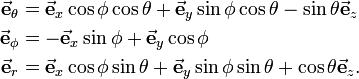\begin{align} \vec\mathbf{e}_\theta &= \vec\mathbf{e}_x \cos\phi\cos\theta + \vec\mathbf{e}_y\sin\phi\cos\theta - \sin\theta \vec\mathbf{e}_z \\ \vec\mathbf{e}_\phi &= -\vec\mathbf{e}_x \sin\phi + \vec\mathbf{e}_y\cos\phi \\ \vec\mathbf{e}_r &= \vec\mathbf{e}_x \cos\phi\sin\theta + \vec\mathbf{e}_y\sin\phi\sin\theta + \cos\theta \vec\mathbf{e}_z . \\ \end{align}

Inverting this set of equations is very easy, since rotation matrices are orthogonal, that is, their inverse is equal to their transpose.

Apparently$\vec{\mathbf{r}}$ is along$\vec{\mathbf{e}}_r$. Since the two rotation matrices are orthogonal (have orthonormal rows and columns), the new frame is orthogonal. Since the two rotation matrices have unit determinant (are proper rotations), the new frame is right-handed.

Recall, parenthetically, that free parallel vectors of equal length have the same coordinate triplet with respect to a given coordinate frame. Or, equivalently, coordinate frames may be freely translated in a parallel manner. That is, the frame in the figure could have been drawn equally well with its origin in the crossing of the x-, y-, and z-axes, which, however, would have obscured the fact that$\vec{\mathbf{e}}_\phi$ and$\vec{\mathbf{e}}_\theta$ are tangent to the surface of the sphere.

## Metric tensor

In curvilinear coordinates q i the metric tensor (with elements g ij ) defines the square of an infinitesimal distance,$ds^2 \equiv \sum_{i=1}^3 g_{ij} dq^i dq^j.$

The Cartesian metric tensor is the identity matrix and hence in Cartesian coordinates,$ds^2 = dx^2 + dy^2 + dz^2 = (dx\, dy\, dz) \begin{pmatrix} dx\\ dy \\ dz\end{pmatrix}.$

Consider the following expressions between differentials, obtained by application of the chain rule,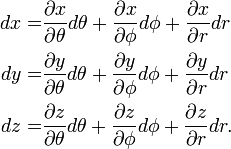\begin{align} dx =& \frac{\partial x}{\partial \theta} d\theta + \frac{\partial x}{\partial \phi}d\phi+ \frac{\partial x}{\partial r} dr \\ dy =& \frac{\partial y}{\partial \theta} d\theta + \frac{\partial y}{\partial \phi}d\phi+ \frac{\partial y}{\partial r} dr \\ dz =& \frac{\partial z}{\partial \theta} d\theta + \frac{\partial z}{\partial \phi} d\phi +\frac{\partial z}{\partial r} dr . \\ \end{align}

In matrix form,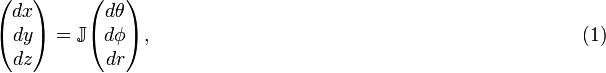$\begin{pmatrix} dx \\ dy \\ dz \\ \end{pmatrix} = \mathbb{J} \begin{pmatrix} d\theta \\ d\phi \\ dr \\ \end{pmatrix}, \qquad\qquad\qquad\qquad\qquad\qquad\qquad\qquad\qquad\qquad\qquad (1)$

with the Jacobi matrix having the following form,$\mathbb{J} \equiv \begin{pmatrix} \frac{\partial x}{\partial \theta} & \frac{\partial x}{\partial \phi}& \frac{\partial x}{\partial r} \\ \frac{\partial y}{\partial \theta} & \frac{\partial y}{\partial \phi}& \frac{\partial y}{\partial r} \\ \frac{\partial z}{\partial \theta} & \frac{\partial z}{\partial \phi} &\frac{\partial z}{\partial r} \\ \end{pmatrix} = \begin{pmatrix} r\cos\phi\cos\theta & - r\sin\phi\sin\theta & \cos\phi\sin\theta \\ r\sin\phi\cos\theta & r\cos\phi\sin\theta & \sin\phi\sin\theta \\ -r\sin\theta & 0 & \cos\theta \\ \end{pmatrix},$

where the matrix on the right-hand side is obtained by performing the partial derivations. By inspection it follows that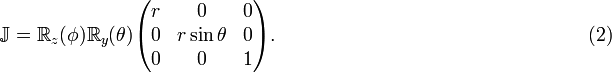$\mathbb{J} = \mathbb{R}_z(\phi) \mathbb{R}_y(\theta) \begin{pmatrix} r & 0 & 0 \\ 0 & r\sin\theta & 0 \\ 0 & 0 & 1 \\ \end{pmatrix}. \qquad \qquad\qquad\qquad\qquad\qquad\qquad\qquad (2)$

The columns of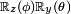$\scriptstyle\mathbb{R}_z(\phi) \mathbb{R}_y(\theta)$ are orthogonal vectors that are normalized to unity. Hence the columns of the Jacobi matrix, which are proportional to the columns of$\scriptstyle\mathbb{R}_z(\phi) \mathbb{R}_y(\theta)$, are orthogonal, but not normalized. The inverses of the normalization factors are on the diagonal of the matrix on the right of the expression. These inverse normalization factors are known as scale factors or Lamé factors. Usually they are denoted by h. Hence the spherical polar scale factors are$h_\theta = r, \qquad h_\phi = r\sin\theta, \qquad h_r = 1.$

The infinitesimal distance squared can be written as follows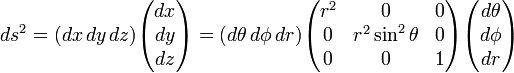$ds^2 = (dx\, dy\, dz) \begin{pmatrix} dx\\ dy \\ dz\end{pmatrix} = (d\theta\, d\phi\, dr) \begin{pmatrix} r^2 & 0 & 0 \\ 0 & r^2\sin^2\theta & 0 \\ 0 & 0 & 1 \\ \end{pmatrix}\begin{pmatrix} d\theta \\ d\phi \\ dr \end{pmatrix}$

where we used that the rotation matrices are orthogonal (matrix times its transpose gives the identity matrix), so that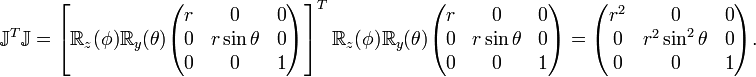$\mathbb{J}^T\mathbb{J} = \left[ \mathbb{R}_z(\phi) \mathbb{R}_y(\theta) \begin{pmatrix} r & 0 & 0 \\ 0 & r\sin\theta & 0 \\ 0 & 0 & 1 \\ \end{pmatrix} \right]^T \mathbb{R}_z(\phi) \mathbb{R}_y(\theta) \begin{pmatrix} r & 0 & 0 \\ 0 & r\sin\theta & 0 \\ 0 & 0 & 1 \\ \end{pmatrix} = \begin{pmatrix} r^2 & 0 & 0 \\ 0 & r^2\sin^2\theta & 0 \\ 0 & 0 & 1 \\ \end{pmatrix}.$

The rightmost matrix being the metric tensor associated with spherical polar coordinates, we find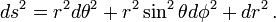$ds^2 = r^2 d\theta^2 + r^2\sin^2\theta d\phi^2 + dr^2 \,.$

The fact that the metric tensor is diagonal is expressed by stating that the spherical polar coordinate system is orthogonal. We see that the metric tensor has the squares of the respective scale factors on the diagonal.

## Infinitesimal surface and volume element

An infinitesimal surface element spanned by two coordinates is equal to the square root of the corresponding 2 × 2 minor (subdeterminant) of the metric tensor times the infinitesimal coordinates,$dA^{(ik)} = \sqrt{g_{ii}g_{kk} - g_{ik}^2} \, dq^{i} dq^{k}.$

For spherical polar coordinates it follows that\begin{align} dA^{(\theta \phi)} &= r^2 \sin\theta\, d\theta d\phi \\ dA^{(r \theta)} &= r\, dr d\theta \\ dA^{(\phi r)} &= r \sin\theta d\phi dr \\ \end{align}

As an example we compute the area of the surface of a sphere with radius r,$A= \int_{0}^\pi \int_{0} ^{2\pi} r^2\sin\theta\, d\theta d\phi = 4\pi r^2.$

The weight appearing in the infinitesimal volume element dV is the Jacobian (the determinant of the Jacobi matrix, which is the square root of the determinant of the metric tensor),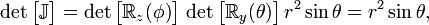$\det\big[\mathbb{J}\big] = \det\big[\mathbb{R}_z(\phi)\big]\,\det\big[\mathbb{R}_y(\theta)\big] \, r^2\sin\theta = r^2\sin\theta,$

where we used that the determinant of a diagonal matrix is the product of its diagonal elements and the fact that the determinants of proper rotation matrices are unity. Because hr = 1, it so happens that the weight of the volume element is equal to the weight of the surface element perpendicular to r.

As an example of the use of dV, the volume V of a sphere with radius R is,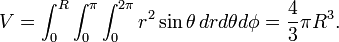$V= \int_{0}^R \int_{0}^\pi \int_{0} ^{2\pi} r^2\sin\theta\, dr d\theta d\phi =\frac{4}{3}\pi R^3.$

## Velocity

We will express the velocity of a particle in spherical polar coordinates. In Cartesian coordinates velocity is the vector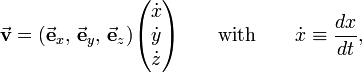$\vec{\mathbf{v}} = (\vec\mathbf{e}_x, \, \vec\mathbf{e}_y, \, \vec\mathbf{e}_z) \begin{pmatrix} \dot{x} \\ \dot{y} \\ \dot{z} \\ \end{pmatrix}\qquad\hbox{with}\qquad \dot{x} \equiv \frac{dx}{dt},$

and similarly the time derivatives of y, z , θ, φ, and r are given in Newton's fluxion (dot) notation. The following two equations follow from Eqs. (1) and (2), respectively,\begin{align} \vec{\mathbf{v}} = (\vec\mathbf{e}_x, \, \vec\mathbf{e}_y, \, \vec\mathbf{e}_z) \mathbb{J} \begin{pmatrix} \dot{\theta} \\ \dot{\phi} \\ \dot{r} \\ \end{pmatrix} = (\vec\mathbf{e}_x, \, \vec\mathbf{e}_y, \, \vec\mathbf{e}_z) \mathbb{R}_z(\phi) \mathbb{R}_y(\theta) \begin{pmatrix} r & 0 & 0 \\ 0 & r\sin\theta & 0 \\ 0 & 0 & 1 \\ \end{pmatrix} \begin{pmatrix} \dot{\theta} \\ \dot{\phi} \\ \dot{r} \\ \end{pmatrix}. \end{align}

Recalling that the unit spherical polar vectors are obtained by this rotation, we find$\vec{\mathbf{v}} = (\vec\mathbf{e}_\theta, \, \vec\mathbf{e}_\phi, \, \vec\mathbf{e}_r) \begin{pmatrix} r & 0 & 0 \\ 0 & r\sin\theta & 0 \\ 0 & 0 & 1 \\ \end{pmatrix} \begin{pmatrix} \dot{\theta} \\ \dot{\phi} \\ \dot{r} \\ \end{pmatrix}$,

so that the velocity expressed in spherical polar coordinates becomes$\vec{\mathbf{v}} = \vec\mathbf{e}_\theta\, r\dot{\theta} + \vec\mathbf{e}_\phi\, r\sin\theta\dot{\phi} +\vec\mathbf{e}_r\, \dot{r}.$

Note parenthetically that$\vec{\mathbf{v}}\cdot\vec{\mathbf{v}} = \Big(\frac{ds}{dt}\Big)^2 = r^2\dot{\theta}^2 + r^2\sin^2\theta\dot{\phi}^2 + \dot{r}^2.$

which becomes upon multiplication by dt2 the expression for ds2 given above.

## Differential operators

In vector analysis a number of differential operators expressed in curvilinear coordinates play an important role. They are the gradient, the divergence, the curl, and the Laplace operator. It is possible to derive general expressions for these operators that are valid in any coordinate system and are based on the metric tensor associated with the coordinate system. In the case of orthogonal systems (diagonal metric tensors) only the square roots of the diagonal elements (the scale factors) appear in the expressions. Since these general relations exist, we will not give derivations for the special case of spherical polar coordinates, but depart from the general expressions.

Above we derived the following scale factors for the spherical polar coordinates,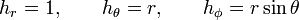$h_r = 1, \qquad h_\theta = r, \qquad h_\phi = r\sin\theta$

and we showed that the unit vectors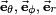$\vec\mathbf{e}_\theta, \, \vec\mathbf{e}_\phi, \, \vec\mathbf{e}_r$ are obtained by two rotations of a Cartesian system.

The gradient of a scalar function Φ is,$\nabla \Phi = \frac{\partial \Phi}{\partial r}\vec\mathbf{e}_r +\frac{1}{r} \frac{\partial \Phi}{\partial \theta}\vec\mathbf{e}_\theta +\frac{1}{r\sin\theta} \frac{\partial \Phi}{\partial \phi}\vec\mathbf{e}_\phi.$

If the vector function A is,$\mathbf{A} = A_r \vec\mathbf{e}_r + A_\theta\vec\mathbf{e}_\theta +A_\phi\vec\mathbf{e}_\phi.$

then its divergence is,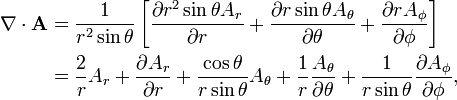\begin{align} \nabla \cdot \mathbf{A} &= \frac{1}{r^2\sin\theta}\left[ \frac{\partial r^2\sin\theta A_r}{\partial r} +\frac{\partial r\sin\theta A_\theta }{\partial \theta} + \frac{\partial r A_\phi}{\partial \phi}\right] \\ & = \frac{2}{r} A_r + \frac{\partial A_r}{\partial r} + \frac{\cos\theta}{r\sin\theta} A_\theta + \frac{1}{r}\frac{A_\theta }{\partial \theta}+ \frac{1}{r\sin\theta}\frac{\partial A_\phi}{\partial \phi}, \end{align}

and its curl is given by\begin{align} \nabla \times \mathbf{A} &= \frac{1}{r^2\sin\theta} \left( \frac{\partial}{\partial \theta} (r\sin\theta A_\phi) - \frac{\partial}{\partial \phi} (r A_\theta) \right) \vec\mathbf{e}_r \\ &\quad + \frac{1}{r\sin\theta} \left( \frac{\partial}{\partial \phi} A_r - \frac{\partial}{\partial r} (r\sin\theta A_\phi) \right) \vec\mathbf{e}_\theta \\ &\quad + \frac{1}{r} \left( \frac{\partial}{\partial r} (r A_\theta) - \frac{\partial}{\partial \theta} A_r \right) \vec\mathbf{e}_\phi. \end{align}

This is often written as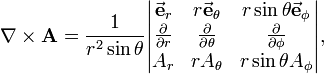$\nabla \times \mathbf{A} = \frac{1}{r^2\sin\theta} \begin{vmatrix} \vec\mathbf{e}_r & r\vec\mathbf{e}_\theta & r\sin\theta\vec\mathbf{e}_\phi \\ \frac{\partial}{\partial r} & \frac{\partial}{\partial \theta} & \frac{\partial}{\partial \phi} \\ A_r & r A_\theta & r\sin\theta A_\phi \\ \end{vmatrix},$

where it is understood that the determinant is computed by developing along the first row and then along the second row.

The Laplace operator of the scalar function Φ is,$\nabla^2 \Phi = \frac{1}{r^2}\left[\frac{\partial }{\partial r} r^2 \frac{\partial \Phi}{\partial r} + \frac{1}{\sin\theta} \frac{\partial}{\partial\theta} \sin\theta \frac{\partial \Phi}{\partial \theta} + \frac{1}{\sin^2\theta} \frac{\partial^2 \Phi}{\partial\phi^2} \right].$

Note that$\frac{1}{r^2}\frac{\partial }{\partial r} r^2 \frac{\partial \Phi}{\partial r} = \frac{1}{r} \frac{\partial^2 r \Phi}{\partial r^2}.$